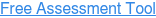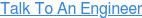###### There are countless ways to take advantage of BlackDiamond Studio and SnowConvert.We Are GAP Mobilize# Migration of ByRef / ByVal parameters

In VB6, by default, parameters are passed by reference. So it’s common, when writing VB6 code not to worry about this. As a result most parameters will end up as ByRef without explicitly declaring them that way.

In VB6 ByRef arguments can take constant values, literals, invocations and other expressions that are not supported in C#.

VBUC corrects this conflict between C# and VB6 by generating auxiliary variables:

`int tempRefParam = 1;Foo(ref tempRefParam);`

To alleviate this issue, the new VBUC will thoroughly analyze the source code to determine if a parameter can be safely converted from ByRef to ByVal.

This improvement will, in typical code, reduce by 70% the quantity of temporary variables generated by the VBUC. The generate code will be more readable and maintainable, and also will reduce the number of compilation errors.

### VB6 COde

`Public Sub F1(p1 As Integer, p2 As Integer)        F1A(p1, p2)    End Sub    Public Sub F2(p1 As Integer, p2 As Integer)        F2A(p1, p2)    End Sub    Public Sub F1A(p1 As Integer, p2 As Integer)        p2 = p2 + p1        MsgBox("p1=" & p1 & " p2=" & p2)    End Sub    Public Sub F2A(p1 As Integer, p2 As Integer)        MsgBox("p1=" & p1 & " p2=" & p2)    End Sub    Private Sub Form_Load()        F1(1, 1)        F2(2, 2)    End Sub`

### .NET Code

`public void  F1( int p1, ref  int p2){     F1A(p1, ref p2);}public void  F2( int p1,  int p2){     F2A(p1, p2);}Public void  F1A( int p1, ref  int p2){     p2 += p1;     MessageBox.Show(“p1=“ + p1.ToString() + “ p2=“ + p2.ToString(),      Application.ProductName);}Public void  F2A( int p1,  int p2){     MessageBox.Show(“p1=“ + p1.ToString() + “ p2=“ + p2.ToString(),      Application.ProductName);}Private void  Form1_Load( Object eventSender,  EventArgs eventArgs){     int tempRefParam = 1;     F1(1, ref tempRefParam);     F2(2, 2);} `
• In function F1, parameter p2 cannot be changed to ByVal because this parameter is modified in function F1A
• All the other parameters were safely migrated as ByVal since they are not modified within the function’s scope